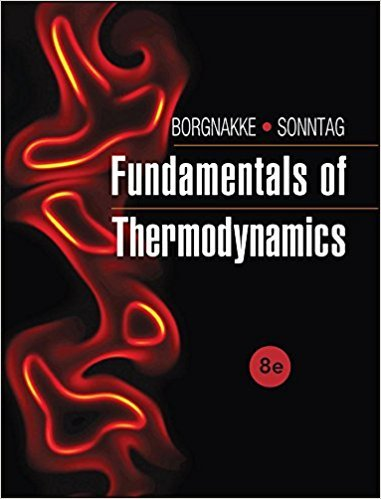×
Get Full Access to Fundamentals Of Thermodynamcs - 8 Edition - Chapter 3 - Problem 131hp
Get Full Access to Fundamentals Of Thermodynamcs - 8 Edition - Chapter 3 - Problem 131hp

×

# Nitrogen at 300 K, 3 MPa is heated to 500 K. Find theISBN: 9781118131992 58

## Solution for problem 131HP Chapter 3

Fundamentals of Thermodynamcs | 8th Edition

• Textbook Solutions
• 2901 Step-by-step solutions solved by professors and subject experts
• Get 24/7 help from StudySoup virtual teaching assistantsFundamentals of Thermodynamcs | 8th Edition

4 5 1 350 Reviews
19
3
Problem 131HP

Nitrogen at 300 K, 3 MPa is heated to 500 K. Find the change in enthalpy using (a) TableB.6, (b) Table A.8, and (c) Table A.5.

Step-by-Step Solution:

Solution 131HP

Step 1 of 5

The objective here is to calculate the change in enthalpy of Nitrogen from the given data.

Step 2 of 5

Step 3 of 5

##### ISBN: 9781118131992

Unlock Textbook Solution